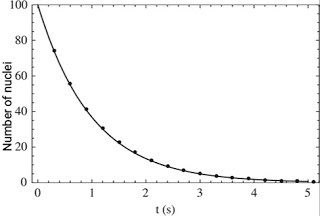# Law of Radioactive Disintegration | Half Life

According to the law of radioactive Disintegration... Units of Activity:- Becquerel, Curie (Ci), rutherford (Rd), Half-life, radioactivity

According to the law of radioactive disintegration the rate of spontaneous disintegration of a radioactive element is proportional to the number of nuclei present at that time.

Mathematically, it can be written as

\frac{dN}{dt}\infty N................(1)

where, N is the number of atoms present at time t. Removing proportionality sign, we get

\frac{dN}{dt}=-\lambda N............(2)

where, λ is a constant of proportionality and is known as decay constant of the element. Negative sign indicates that as t increase N decreases.

\frac{dN}N=-\lambda dt.............(3)

Integrating both sides, we have

\int\frac{dN}N=-\lambda\int dt

\log_e(N)=-\lambda t+C.............(4)

where C is constant of integration and is evaluated by the fact that at t = 0, number of atoms of the radioactive element is N0. Using this condition, we get

C=\log_e(N_0).................(5)

Substituting this value of C in Eq. (5), we get

\log_e(N)=-\lambda t+\log_e(N_0)

\log_e(N)-\log_e(N_0)=-\lambda t

Thus,

N=N_0e^{-\lambda t}.................(6)Exponential decay curve

## Units of Activity

The SI unit of activity is named after Becquerel. Active of a radioactive sample is said to be 1 becquerel if rate of decay is 1 nucleus per second.

Therefore,

becquerel = 1 Bq = 1 decay/sec

An older unit Curie (Ci) is still commonly used to measure activity.

Therefore,

1 curie = 1 Ci = 3.7 × 10^{10} decay/sec = 3.7 × 10^{10} Bq

Yet, another unit of activity of radioactive sample is rutherford (Rd) and is defined as :

1 rutherford = 1 Rd = 10^{10} decay/sec = 10^{10} Bq

## Half-life

Half-life is the time at which the activity of sample has been reduced to one-half of its initial value.

If N0 is the initial number of radioactive nuclei present, then in one half-life this number will reduce to N0/2. Therefore,

N=\frac{N_0}2

substituting N in Eq. (6), we get

\frac{N_0}2=N_0e^{-\lambda t_{1/2}}

\1/2=e^{-\lambda t_{1/2}}

e^{\lambda t_{1/2}}=2

Taking natural log on both sides,

\lambda t_{1/2}=\log_e2

t_{1/2}=\frac{2.303\log_{10}\2}\lambda

[ \log_ex=2.303\log_{10}x\ ]

t_{1/2}=\frac{0.693}\lambda

Therefore, half-life of a radioactive material depends upon the disintegration constant. The larger the value of disintegration constant, smaller is the half-life. Half-life of a radioactive material is its intrinsic property it cannot be altered by any physical or chemical means.